# Evaluating Exponential and Logarithmic Functions: Homework Help Chapter Exam

Exam Instructions:

Choose your answers to the questions and click 'Next' to see the next set of questions. You can skip questions if you would like and come back to them later with the yellow "Go To First Skipped Question" button. When you have completed the practice exam, a green submit button will appear. Click it to see your results. Good luck!

### Page 2

#### Question 7 7. Solve, to 2 decimal places, the exponential equation below for x: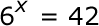#### Question 8 8. Evaluate the logarithm below: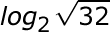#### Question 9 9. Expand the following logarithm: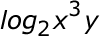### Page 3

#### Question 12 12. Use the following information to answer the question.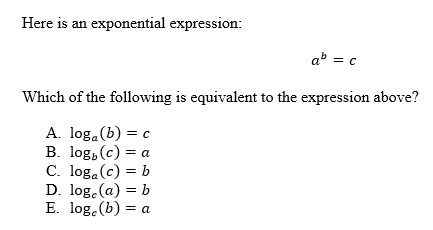### Page 4

#### Question 17 17. Solve the exponential equation below for x: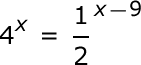#### Question 19 19. Solve the exponential equation below for x: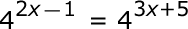#### Question 20 20. Evaluate the following.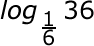### Page 5

#### Question 21 21. Evaluate the logarithm below to 2 decimal places.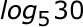#### Question 22 22. Evaluate the following.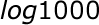#### Question 24 24. Solve the following equation for x.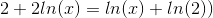### Page 6

#### Question 26 26. Condense the logarithm below: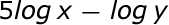#### Question 27 27. Which logarithmic property is shown below?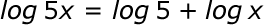#### Question 28 28. Evaluate the following.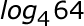#### Question 29 29. Solve the exponential equation below for x: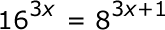#### Question 30 30. Which equation represents this graph?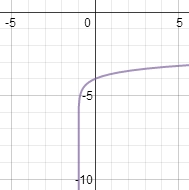#### Evaluating Exponential and Logarithmic Functions: Homework Help Chapter Exam Instructions

Choose your answers to the questions and click 'Next' to see the next set of questions. You can skip questions if you would like and come back to them later with the yellow "Go To First Skipped Question" button. When you have completed the practice exam, a green submit button will appear. Click it to see your results. Good luck!

Support